• +91 9971497814
• info@interviewmaterial.com

# Chapter 2- बहुपद (Polynomials) Ex-2.3 Interview Questions Answers

### Related Subjects

Question 1 :
विभाजन एल्गोरिथम का प्रयोग करके, निम्न में p(x) को g(x) से भाग देने पर भागफल तथा शेषफल ज्ञात कीजिए :
(i) p(x) = x3 – 3x2 + 5x – 3, g(x) = x2 – 2
(ii) p(x) = x4 – 3x2 + 4x + 5, g(x) = x2 + 1 – x
(iii) p(x) = x4 – 5x + 6, g(x) = 2 – x2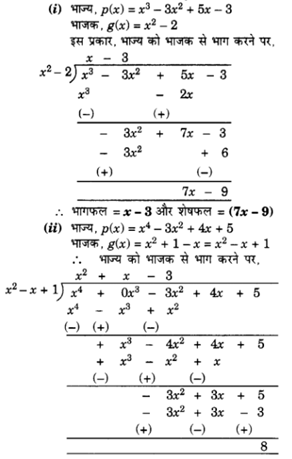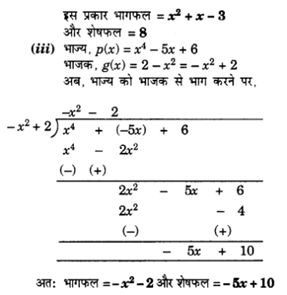Question 2 :
पहले बहुपद से दुसरे बहुपद को भाग करके, जाँच कीजिए कि क्या प्रथम बहुपद द्वितीय का एक गुणनखंड है :
(i) t2 – 3, 2t4 + 3t3 – 2t2 – 9t – 12
(ii) x2 + 3x + 1, 3x4 + 5x3 – 7x2 + 2x + 2
(iii) x3 – 3x + 1, x5 – 4x3 + x2 + 3x + 1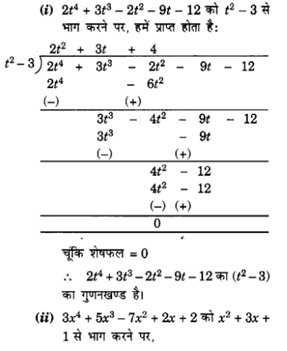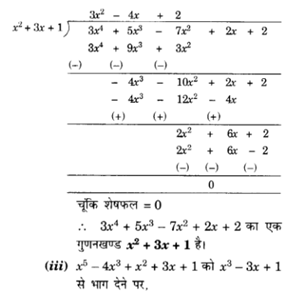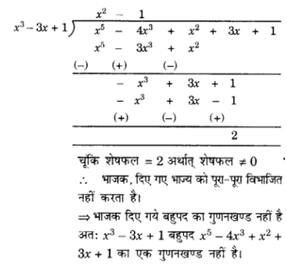Question 3 :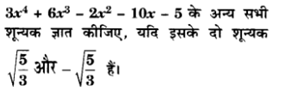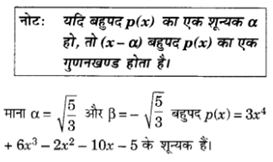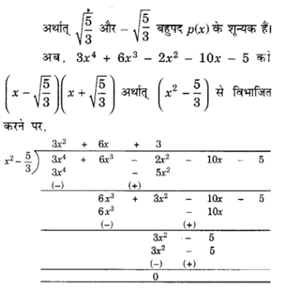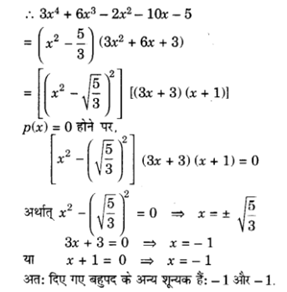Question 4 : यदि x3 – 3x2 + x + 2 को एक बहुपद g(x) से भाग देने पर, भागफल और शेषफल क्रमश: x – 2 और – 2x + 4 हैं तो g(x) ज्ञात कीजिए।

Answer 4 : दिया है : भाज्य p(x)= x3 – 3x2 + x + 2
भागफलq(x) = x – 2,
शेषफलr(x) = -2x + 4
भाजकg(x) = ?
भाज्य = भाजक × भागफल + शेषफल
p(x) = g(x) × q(x) + r(x)
x3 – 3x2 + x + 2 = g(x) (x – 2) + (- 2x + 4)
x3 – 3x2 + x + 2 + 2x – 4 = g(x) (x – 2)
g(x) (x – 2) = x3 – 3x2 + 3x – 2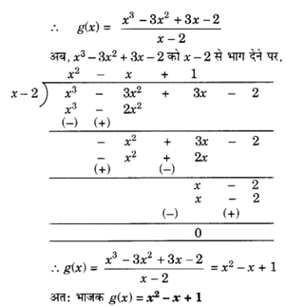Question 5 :
बहुपदों p(x), g(x), q(x) और r(x) के ऐसे उदाहरण दीजिए जो विभाजन एल्गोरिथम को संतुष्ट करते हों तथा
(i) घात p(x) = घात q(x) हो
(ii) घात q(x) = घात r(x) हो
(iii) घात r(x) = 0

Answer 5 : विभाजन एल्गोरिथ्म को संतुष्ट करते हुए प्रत्येक का एक-एक उदाहरण इस प्रकार हैः ।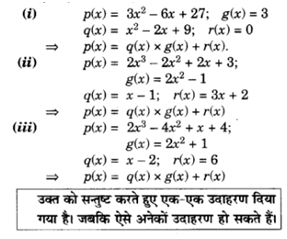Todays Deals### Chapter 2- बहुपद (Polynomials) Ex-2.3 Contributorskrishan

Name:
Email:

# Latest News# 9000 interview questions in different categories